# Logistic Regression with Python¶

## Import Libraries¶

Let's import some libraries to get started!

In :
import numpy as np
import pandas as pd
import matplotlib.pyplot as plt
import seaborn as sns

%matplotlib inline
sns.set_style('whitegrid')


## The Data¶

We will be working with the Titanic Data Set from Kaggle downloaded as titanic_train.csv file

In :
train = pd.read_csv('titanic_train.csv')


Out:
PassengerId Survived Pclass Name Sex Age SibSp Parch Ticket Fare Cabin Embarked
0 1 0 3 Braund, Mr. Owen Harris male 22.0 1 0 A/5 21171 7.2500 NaN S
1 2 1 1 Cumings, Mrs. John Bradley (Florence Briggs Th... female 38.0 1 0 PC 17599 71.2833 C85 C

# Exploratory Data Analysis¶

Some exploratory data analysis!

We'll start by checking out missing data!

## Missing Data¶

We can use seaborn to create a simple heatmap to see where we are missing data!

In :
sns.heatmap(train.isnull(),yticklabels=False,cbar=False,cmap='viridis')

# An assessment of data available, Age and Cabin have missing values while the rest
# are relatively OK.

Out:
<matplotlib.axes._subplots.AxesSubplot at 0x1a208d1908>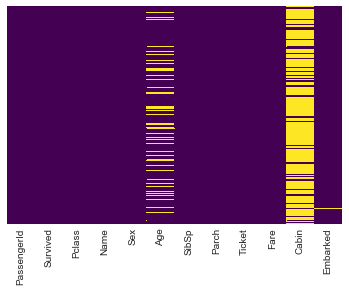#### Visualizing some more of the data¶

analysis by column. By Survival

In :
sns.countplot(x='Survived',data=train)

Out:
<matplotlib.axes._subplots.AxesSubplot at 0x1a20274080>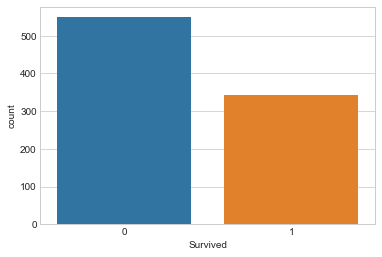#### Survival by Gender¶

In :
sns.countplot(x='Survived',hue='Sex',data=train,palette='RdBu_r')

Out:
<matplotlib.axes._subplots.AxesSubplot at 0x1a20d895c0>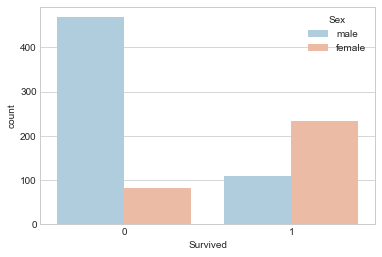#### Survival by Passenger Class¶

In :
sns.countplot(x='Survived',hue='Pclass',data=train)

Out:
<matplotlib.axes._subplots.AxesSubplot at 0x1a20976320>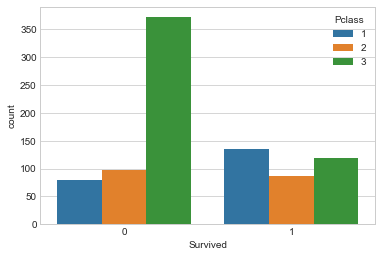#### Distribution of Passengers on board by Age¶

In :
sns.distplot(train['Age'].dropna(),kde=False,bins=30)

Out:
<matplotlib.axes._subplots.AxesSubplot at 0x1a20e2ac18>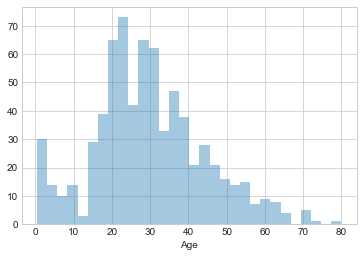#### Passengers onboard with sibling(s) / spouse¶

In :
sns.countplot(x='SibSp',data=train)

Out:
<matplotlib.axes._subplots.AxesSubplot at 0x1a20ede5c0>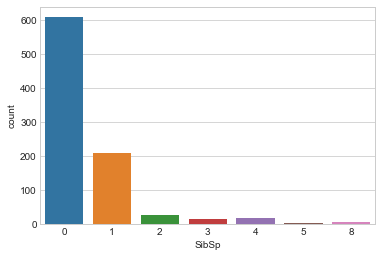#### Passengers by amount of fare paid¶

In :
train['Fare'].hist(bins=20,figsize=(10,5))

Out:
<matplotlib.axes._subplots.AxesSubplot at 0x1a210737b8>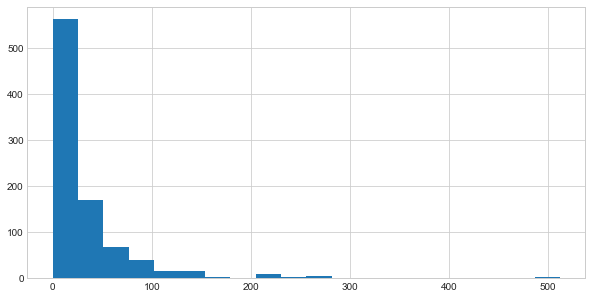# Data Cleaning¶

#### Imputation.¶

Filling out missing values by approximation


Filling in the mean age to the age column

Start of by checking the average age by passenger class.

In :
plt.figure(figsize=(10,7))
sns.boxplot(x='Pclass',y='Age',data=train)

Out:
<matplotlib.axes._subplots.AxesSubplot at 0x1a20ff03c8>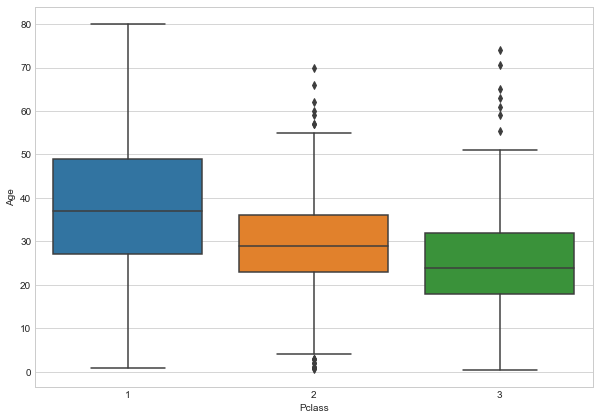Wealthier passengers in the higher classes tend to be older,

We'll use these average age values to impute missing data based on Pclass for Age.

In :
def impute_age(cols):
Age = cols
Pclass = cols

if pd.isnull(Age):
if Pclass == 1:
return 37
elif Pclass == 2:
return 29
else:
return 24

else:
return Age



Apply impute_age function

In :
train['Age'] = train[['Age','Pclass']].apply(impute_age,axis=1)


And by checking for missing values on our data, we have;

In :
sns.heatmap(train.isnull(),yticklabels=False,cbar=False,cmap='viridis')

Out:
<matplotlib.axes._subplots.AxesSubplot at 0x1a212b75f8>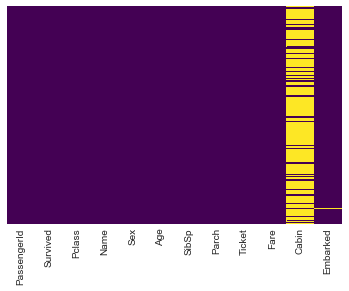We can Drop the Cabin column as it possesses a huge percentage of missing values and filling in may not be appropriatte.

Also we will drop the few instances on the Embarked column

In :
train.drop('Cabin',axis=1,inplace=True)

In :
train.head(2)

Out:
PassengerId Survived Pclass Name Sex Age SibSp Parch Ticket Fare Embarked
0 1 0 3 Braund, Mr. Owen Harris male 22.0 1 0 A/5 21171 7.2500 S
1 2 1 1 Cumings, Mrs. John Bradley (Florence Briggs Th... female 38.0 1 0 PC 17599 71.2833 C
In :
#drop missing row record from embarked column
train.dropna(inplace=True)


## Convert Categorical Features¶

We need to convert categorical features to dummy variables using pandas,

Otherwise the learning algorithm won't be able to directly take in those features as inputs.

For the sex column, caterorize if passenger is male or not(1 | 0 )

On embarkment point it will be Q, S 0r C.

In :
sex = pd.get_dummies(train['Sex'],drop_first=True)

embark = pd.get_dummies(train['Embarked'],drop_first=True)


#### Concatenate the generated categorical columns to the dataset¶

In :
train = pd.concat([train, sex,embark],axis=1)

Out:
PassengerId Survived Pclass Name Sex Age SibSp Parch Ticket Fare Embarked male Q S
0 1 0 3 Braund, Mr. Owen Harris male 22.0 1 0 A/5 21171 7.2500 S 1 0 1
1 2 1 1 Cumings, Mrs. John Bradley (Florence Briggs Th... female 38.0 1 0 PC 17599 71.2833 C 0 0 0

#### Select Columns that we will use for the model¶

In :
train.drop(['Name','Sex','Embarked','Ticket','PassengerId'],axis=1,inplace=True)

In :
train.head(2)

Out:
Survived Pclass Age SibSp Parch Fare male Q S
0 0 3 22.0 1 0 7.2500 1 0 1
1 1 1 38.0 1 0 71.2833 0 0 0

And the data is ready for our model!

# Building a Logistic Regression model¶

Start by splitting data into a training set and test set

## Train Test Split¶

X = These are the features we will use to predict

y = Value we are predicting ie Did the passenger survive
In :
X = train.drop('Survived',axis=1)
y = train['Survived']

In :
from sklearn.cross_validation import train_test_split

In :
X_train, X_test, y_train, y_test = train_test_split(X, y, test_size=0.3, random_state=101)


## Training and Predicting¶

In :
from sklearn.linear_model import LogisticRegression

In :
# create an instance of LR model
logmodel = LogisticRegression()

In :
# train the model

logmodel.fit(X_train,y_train)

Out:
LogisticRegression(C=1.0, class_weight=None, dual=False, fit_intercept=True,
intercept_scaling=1, max_iter=100, multi_class='ovr', n_jobs=1,
penalty='l2', random_state=None, solver='liblinear', tol=0.0001,
verbose=0, warm_start=False)
In :
# predict using the model
predictions = logmodel.predict(X_test)


## Evaluate the Model¶

Using classification report, We can check :

- precision
- recall
- f1-score 
In :
from sklearn.metrics import classification_report,confusion_matrix

In :
print(classification_report(y_test,predictions))

             precision    recall  f1-score   support

0       0.80      0.91      0.85       163
1       0.82      0.65      0.73       104

avg / total       0.81      0.81      0.80       267



A confusion matrix can also be applied

in order to determine how many observations were correctly or incorrectly classified.

In :
confusion_matrix(y_test,predictions)

Out:
array([[148,  15],
[ 36,  68]])